Publicité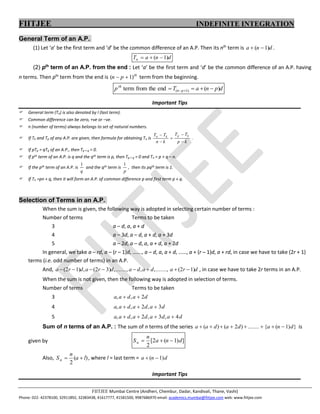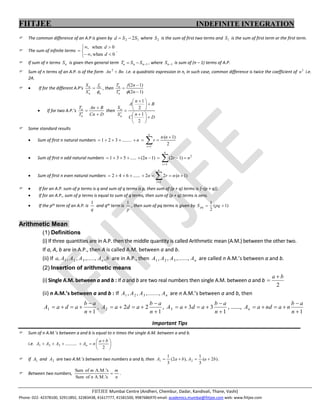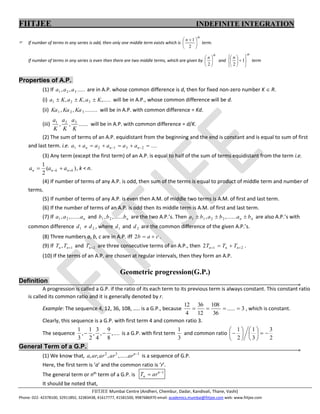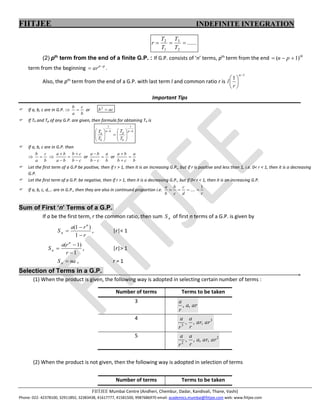Publicité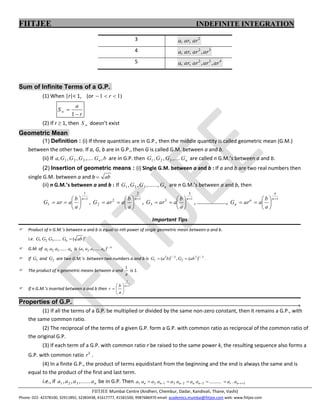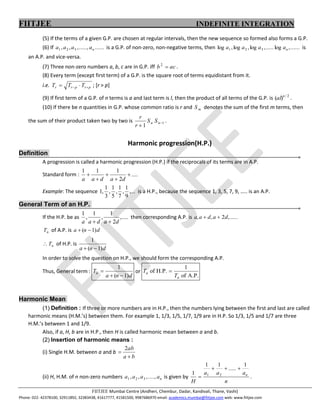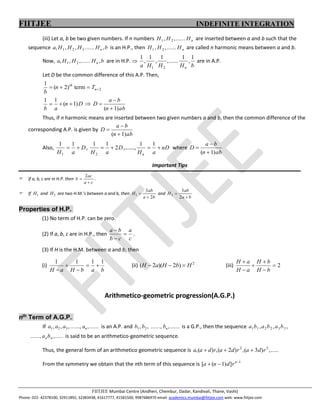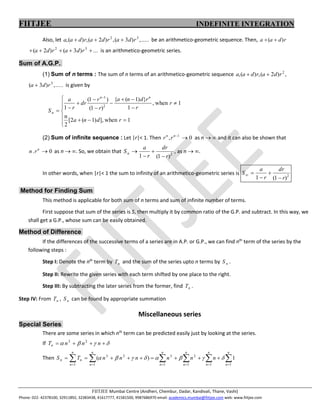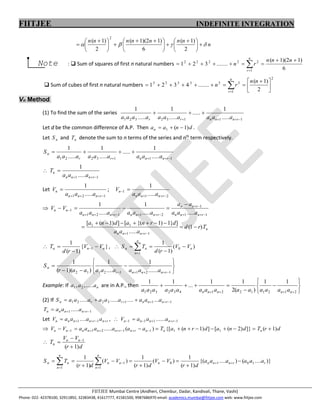Publicité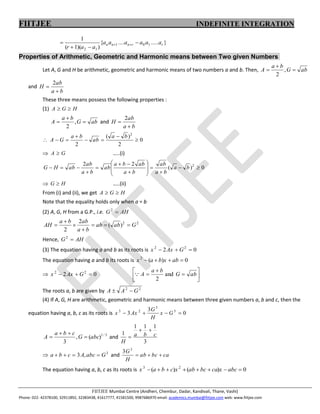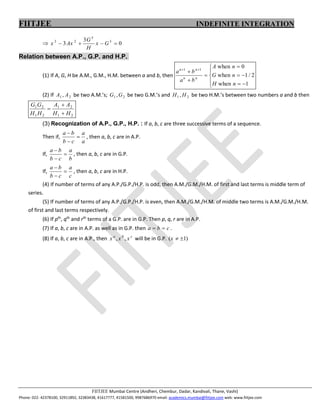Prochain SlideShareProgression & Series-01-Theory.pdf
Chargement dans ... 3
1 sur 11
Publicité

### progressions.docx

1. FIITJEE INDEFINITE INTEGRATION FIITJEE Mumbai Centre (Andheri, Chembur, Dadar, Kandivali, Thane, Vashi) Phone: 022- 42378100, 32911892, 32383438, 41617777, 41581500, 9987686970 email: academics.mumbai@fiitjee.com web: www.fiitjee.com General Term of an A.P.. (1) Let ‘a’ be the first term and ‘d’ be the common difference of an A.P. Then its nth term is d n a ) 1 (   . d n a Tn ) 1 (    (2) pth term of an A.P. from the end : Let ‘a’ be the first term and ‘d’ be the common difference of an A.P. having n terms. Then pth term from the end is th p n ) 1 (   term from the beginning. d p n a T p p n th ) ( end the from term ) 1 (       Important Tips  General term (Tn) is also denoted by l (last term).  Common difference can be zero, +ve or –ve.  n (number of terms) always belongs to set of natural numbers.  If Tk and Tp of any A.P. are given, then formula for obtaining Tn is k p T T k n T T k p k n      .  If pTp = qTq of an A.P., then Tp + q = 0.  If pth term of an A.P. is q and the qth term is p, then Tp + q = 0 and Tn = p + q – n.  If the pth term of an A.P. is q 1 and the qth term is p 1 , then its pqth term is 1.  If Tn =pn + q, then it will form an A.P. of common difference p and first term p + q. Selection of Terms in an A.P.. When the sum is given, the following way is adopted in selecting certain number of terms : Number of terms Terms to be taken 3 a – d, a, a + d 4 a – 3d, a – d, a + d, a + 3d 5 a – 2d, a – d, a, a + d, a + 2d In general, we take a – rd, a – (r – 1)d, ……., a – d, a, a + d, ……, a + (r – 1)d, a + rd, in case we have to take (2r + 1) terms (i.e. odd number of terms) in an A.P. And, d r a d a d a d r a d r a ) 1 2 ( ......., , , ......., , ) 3 2 ( , ) 1 2 (         , in case we have to take 2r terms in an A.P. When the sum is not given, then the following way is adopted in selection of terms. Number of terms Terms to be taken 3 d a d a a 2 , ,   4 d a d a d a a 3 , 2 , ,    5 d a d a d a d a a 4 , 3 , 2 , ,     Sum of n terms of an A.P. : The sum of n terms of the series } ) 1 ( { ....... ) 2 ( ) ( d n a d a d a a         is given by ] ) 1 ( 2 [ 2 d n a n Sn    Also, ) ( 2 l a n Sn   , where l = last term = d n a ) 1 (   Important Tips
2. FIITJEE INDEFINITE INTEGRATION FIITJEE Mumbai Centre (Andheri, Chembur, Dadar, Kandivali, Thane, Vashi) Phone: 022- 42378100, 32911892, 32383438, 41617777, 41581500, 9987686970 email: academics.mumbai@fiitjee.com web: www.fiitjee.com  The common difference of an A.P is given by 1 2 2S S d   where 2 S is the sum of first two terms and 1 S is the sum of first term or the first term.  The sum of infinite terms          0 when , 0 when , d d .  If sum of n terms n S is given then general term 1    n n n S S T , where 1  n S is sum of (n – 1) terms of A.P.  Sum of n terms of an A.P. is of the form Bn An  2 i.e. a quadratic expression in n, in such case, common difference is twice the coefficient of 2 n i.e. 2A.   If for the different A.P’s n n n n f S S    , then ) 1 2 ( ) 1 2 (     n n f T T n n   If for two A.P.’s D Cn B An T T n n     then D n C B n A S S n n                   2 1 2 1  Some standard results  Sum of first n natural numbers 2 ) 1 ( ........ 3 2 1 1           n n r n n r  Sum of first n odd natural numbers 2 1 ) 1 2 ( ) 1 2 ( ..... 5 3 1 n r n n r             Sum of first n even natural numbers           n r n n r n 1 ) 1 ( 2 2 ...... 6 4 2   If for an A.P. sum of p terms is q and sum of q terms is p, then sum of (p + q) terms is {–(p + q)}.  If for an A.P., sum of p terms is equal to sum of q terms, then sum of (p + q) terms is zero.  If the pth term of an A.P. is q 1 and qth term is p 1 , then sum of pq terms is given by ) 1 ( 2 1   pq Spq Arithmetic Mean. (1) Definitions (i) If three quantities are in A.P. then the middle quantity is called Arithmetic mean (A.M.) between the other two. If a, A, b are in A.P., then A is called A.M. between a and b. (ii) If b A A A A a n , ,....., , , , 3 2 1 are in A.P., then n A A A A ......, , , , 3 2 1 are called n A.M.’s between a and b. (2) Insertion of arithmetic means (i) Single A.M. between a and b : If a and b are two real numbers then single A.M. between a and b 2 b a   (ii) n A.M.’s between a and b : If n A A A A ......., , , , 3 2 1 are n A.M.’s between a and b, then 1 1       n a b a d a A , 1 2 2 2       n a b a d a A , 1 3 3 3       n a b a d a A , ……., 1       n a b n a nd a An Important Tips  Sum of n A.M.’s between a and b is equal to n times the single A.M. between a and b. i.e.             2 .......... 3 2 1 b a n A A A A n  If 1 A and 2 A are two A.M.’s between two numbers a and b, then ) 2 ( 3 1 ), 2 ( 3 1 2 1 b a A b a A     .  Between two numbers, n m n m  s A.M.' of Sum s A.M.' of Sum .
3. FIITJEE INDEFINITE INTEGRATION FIITJEE Mumbai Centre (Andheri, Chembur, Dadar, Kandivali, Thane, Vashi) Phone: 022- 42378100, 32911892, 32383438, 41617777, 41581500, 9987686970 email: academics.mumbai@fiitjee.com web: www.fiitjee.com  If number of terms in any series is odd, then only one middle term exists which is th n        2 1 term. If number of terms in any series is even then there are two middle terms, which are given by th n       2 and th n              1 2 term Properties of A.P.. (1) If ..... , , 3 2 1 a a a are in A.P. whose common difference is d, then for fixed non-zero number K  R. (i) ,..... , , 3 2 1 K a K a K a    will be in A.P., whose common difference will be d. (ii) ........ , , 3 2 1 Ka Ka Ka will be in A.P. with common difference = Kd. (iii) ...... , , 3 2 1 K a K a K a will be in A.P. with common difference = d/K. (2) The sum of terms of an A.P. equidistant from the beginning and the end is constant and is equal to sum of first and last term. i.e. .... 2 3 1 2 1         n n n a a a a a a (3) Any term (except the first term) of an A.P. is equal to half of the sum of terms equidistant from the term i.e. ) ( 2 1 k n k n n a a a     , k < n. (4) If number of terms of any A.P. is odd, then sum of the terms is equal to product of middle term and number of terms. (5) If number of terms of any A.P. is even then A.M. of middle two terms is A.M. of first and last term. (6) If the number of terms of an A.P. is odd then its middle term is A.M. of first and last term. (7) If n a a a ...... , , 2 1 and n b b b ...... , , 2 1 are the two A.P.’s. Then n n b a b a b a    ...... , , 2 2 1 1 are also A.P.’s with common difference 2 1 d d  , where 1 d and 2 d are the common difference of the given A.P.’s. (8) Three numbers a, b, c are in A.P. iff c a b   2 . (9) If 1 ,  n n T T and 2  n T are three consecutive terms of an A.P., then 2 1 2     n n n T T T . (10) If the terms of an A.P. are chosen at regular intervals, then they form an A.P. Geometric progression(G.P.) Definition. A progression is called a G.P. if the ratio of its each term to its previous term is always constant. This constant ratio is called its common ratio and it is generally denoted by r. Example: The sequence 4, 12, 36, 108, ….. is a G.P., because 3 ..... 36 108 12 36 4 12     , which is constant. Clearly, this sequence is a G.P. with first term 4 and common ratio 3. The sequence .... , 8 9 , 4 3 , 2 1 , 3 1   is a G.P. with first term 3 1 and common ratio 2 3 3 1 2 1                General Term of a G.P.. (1) We know that, 1 3 2 ..... , , , ,  n ar ar ar ar a is a sequence of G.P. Here, the first term is ‘a’ and the common ratio is ‘r’. The general term or nth term of a G.P. is 1   n n ar T It should be noted that,
4. FIITJEE INDEFINITE INTEGRATION FIITJEE Mumbai Centre (Andheri, Chembur, Dadar, Kandivali, Thane, Vashi) Phone: 022- 42378100, 32911892, 32383438, 41617777, 41581500, 9987686970 email: academics.mumbai@fiitjee.com web: www.fiitjee.com ...... 2 3 1 2    T T T T r (2) pth term from the end of a finite G.P. : If G.P. consists of ‘n’ terms, pth term from the end th p n ) 1 (    term from the beginning p n ar   . Also, the pth term from the end of a G.P. with last term l and common ratio r is 1 1        n r l Important Tips  If a, b, c are in G.P.  b c a b  or ac b  2  If Tk and Tp of any G.P. are given, then formula for obtaining Tn is k p k p k n k n T T T T                    1 1  If a, b, c are in G.P. then  b c a b   c b c b b a b a      or b a c b b a    or b a c b b a     Let the first term of a G.P be positive, then if r > 1, then it is an increasing G.P., but if r is positive and less than 1, i.e. 0< r < 1, then it is a decreasing G.P.  Let the first term of a G.P. be negative, then if r > 1, then it is a decreasing G.P., but if 0< r < 1, then it is an increasing G.P.  If a, b, c, d,… are in G.P., then they are also in continued proportion i.e. r d c c b b a 1 ....     Sum of First ‘n’ Terms of a G.P.. If a be the first term, r the common ratio, then sum n S of first n terms of a G.P. is given by r r a S n n    1 ) 1 ( , |r|< 1 1 ) 1 (    r r a S n n , |r|> 1 na Sn  , r = 1 Selection of Terms in a G.P.. (1) When the product is given, the following way is adopted in selecting certain number of terms : Number of terms Terms to be taken 3 ar a r a , , 4 3 3 , , , ar ar r a r a 5 2 2 , , , , ar ar a r a r a (2) When the product is not given, then the following way is adopted in selection of terms Number of terms Terms to be taken
5. FIITJEE INDEFINITE INTEGRATION FIITJEE Mumbai Centre (Andheri, Chembur, Dadar, Kandivali, Thane, Vashi) Phone: 022- 42378100, 32911892, 32383438, 41617777, 41581500, 9987686970 email: academics.mumbai@fiitjee.com web: www.fiitjee.com 3 2 , , ar ar a 4 3 2 , , , ar ar ar a 5 4 3 2 , , , , ar ar ar ar a Sum of Infinite Terms of a G.P.. (1) When |r|< 1, (or ) 1 1    r r a S    1 (2) If r  1, then  S doesn’t exist Geometric Mean. (1) Definition : (i) If three quantities are in G.P., then the middle quantity is called geometric mean (G.M.) between the other two. If a, G, b are in G.P., then G is called G.M. between a and b. (ii) If b G G G G a n , ,.... , , , 3 2 1 are in G.P. then n G G G G ,.... , , 3 2 1 are called n G.M.’s between a and b. (2) Insertion of geometric means : (i) Single G.M. between a and b : If a and b are two real numbers then single G.M. between a and b ab  (ii) n G.M.’s between a and b : If n G G G G ......, , , , 3 2 1 are n G.M.’s between a and b, then 1 1 1          n a b a ar G , 1 2 2 2          n a b a ar G , 1 3 3 3          n a b a ar G , ……………….., 1          n n n n a b a ar G Important Tips  Product of n G.M.’s between a and b is equal to nth power of single geometric mean between a and b. i.e. n n ab G G G G ) ( ...... 3 2 1   G.M. of n a a a a ...... 3 2 1 is n n a a a a / 1 3 2 1 ) ..... (  If 1 G and 2 G are two G.M.’s between two numbers a and b is 3 / 1 2 2 3 / 1 2 1 ) ( , ) ( ab G b a G   .  The product of n geometric means between a and a 1 is 1.  If n G.M.’s inserted between a and b then 1 1         n a b r Properties of G.P.. (1) If all the terms of a G.P. be multiplied or divided by the same non-zero constant, then it remains a G.P., with the same common ratio. (2) The reciprocal of the terms of a given G.P. form a G.P. with common ratio as reciprocal of the common ratio of the original G.P. (3) If each term of a G.P. with common ratio r be raised to the same power k, the resulting sequence also forms a G.P. with common ratio k r . (4) In a finite G.P., the product of terms equidistant from the beginning and the end is always the same and is equal to the product of the first and last term. i.e., if n a a a a ...... , , , 3 2 1 be in G.P. Then 1 3 2 3 1 2 1 . ..........           r n r n n n n n a a a a a a a a a a
6. FIITJEE INDEFINITE INTEGRATION FIITJEE Mumbai Centre (Andheri, Chembur, Dadar, Kandivali, Thane, Vashi) Phone: 022- 42378100, 32911892, 32383438, 41617777, 41581500, 9987686970 email: academics.mumbai@fiitjee.com web: www.fiitjee.com (5) If the terms of a given G.P. are chosen at regular intervals, then the new sequence so formed also forms a G.P. (6) If ...... ....., , , , 3 2 1 n a a a a is a G.P. of non-zero, non-negative terms, then ...... , log ..... , log , log , log 3 2 1 n a a a a is an A.P. and vice-versa. (7) Three non-zero numbers a, b, c are in G.P. iff ac b  2 . (8) Every term (except first term) of a G.P. is the square root of terms equidistant from it. i.e. p r p r r T T T     ; [r > p] (9) If first term of a G.P. of n terms is a and last term is l, then the product of all terms of the G.P. is 2 / ) ( n al . (10) If there be n quantities in G.P. whose common ratio is r and m S denotes the sum of the first m terms, then the sum of their product taken two by two is 1 1   n n S S r r . Harmonic progression(H.P.) Definition. A progression is called a harmonic progression (H.P.) if the reciprocals of its terms are in A.P. Standard form : .... 2 1 1 1      d a d a a Example: The sequence ,... 9 1 , 7 1 , 5 1 , 3 1 , 1 is a H.P., because the sequence 1, 3, 5, 7, 9, ….. is an A.P. General Term of an H.P.. If the H.P. be as .... , 2 1 , 1 , 1 d a d a a   then corresponding A.P. is ..... , 2 , , d a d a a   n T of A.P. is d n a ) 1 (    n T of H.P. is d n a ) 1 ( 1   In order to solve the question on H.P., we should form the corresponding A.P. Thus, General term : d n a Tn ) 1 ( 1    or A.P. of 1 H.P. of n n T T  Harmonic Mean. (1) Definition : If three or more numbers are in H.P., then the numbers lying between the first and last are called harmonic means (H.M.’s) between them. For example 1, 1/3, 1/5, 1/7, 1/9 are in H.P. So 1/3, 1/5 and 1/7 are three H.M.’s between 1 and 1/9. Also, if a, H, b are in H.P., then H is called harmonic mean between a and b. (2) Insertion of harmonic means : (i) Single H.M. between a and b b a ab   2 (ii) H, H.M. of n non-zero numbers n a a a a ...., , , , 3 2 1 is given by n a a a H n 1 ..... 1 1 1 2 1     .
7. FIITJEE INDEFINITE INTEGRATION FIITJEE Mumbai Centre (Andheri, Chembur, Dadar, Kandivali, Thane, Vashi) Phone: 022- 42378100, 32911892, 32383438, 41617777, 41581500, 9987686970 email: academics.mumbai@fiitjee.com web: www.fiitjee.com (iii) Let a, b be two given numbers. If n numbers n H H H ...... , , 2 1 are inserted between a and b such that the sequence b H H H H a n , ...... , , , 3 2 1 is an H.P., then n H H H ...... , , 2 1 are called n harmonic means between a and b. Now, b H H H a n , ...... , , , 2 1 are in H.P.  b H H H a n 1 , 1 ...... , 1 , 1 , 1 2 1 are in A.P. Let D be the common difference of this A.P. Then, 2 term ) 2 ( 1     n th T n b D n a b ) 1 ( 1 1     ab n b a D ) 1 (    Thus, if n harmonic means are inserted between two given numbers a and b, then the common difference of the corresponding A.P. is given by ab n b a D ) 1 (    Also, D a H   1 1 1 , D a H 2 1 1 2   ,……., nD a Hn   1 1 where ab n b a D ) 1 (    Important Tips  If a, b, c are in H.P. then c a ac b   2 .  If 1 H and 2 H are two H.M.’s between a and b, then b a ab H 2 3 1   and b a ab H   2 3 2 Properties of H.P.. (1) No term of H.P. can be zero. (2) If a, b, c are in H.P., then c a c b b a    . (3) If H is the H.M. between a and b, then (i) b a b H a H 1 1 1 1      (ii) 2 ) 2 )( 2 ( H b H a H    (iii) 2       b H b H a H a H Arithmetico-geometric progression(A.G.P.) nth Term of A.G.P.. If ...... , ......, , , , 3 2 1 n a a a a is an A.P. and ...... , ......, , , 2 1 n b b b is a G.P., then the sequence , , , 3 3 2 2 1 1 b a b a b a ..... , ......, n nb a is said to be an arithmetico-geometric sequence. Thus, the general form of an arithmetico geometric sequence is ..... , ) 3 ( , ) 2 ( , ) ( , 3 2 r d a r d a r d a a    From the symmetry we obtain that the nth term of this sequence is 1 ] ) 1 ( [    n r d n a
8. FIITJEE INDEFINITE INTEGRATION FIITJEE Mumbai Centre (Andheri, Chembur, Dadar, Kandivali, Thane, Vashi) Phone: 022- 42378100, 32911892, 32383438, 41617777, 41581500, 9987686970 email: academics.mumbai@fiitjee.com web: www.fiitjee.com Also, let ..... , ) 3 ( , ) 2 ( , ) ( , 3 2 r d a r d a r d a a    be an arithmetico-geometric sequence. Then, r d a a ) (   ... ) 3 ( ) 2 ( 3 2      r d a r d a is an arithmetico-geometric series. Sum of A.G.P.. (1) Sum of n terms : The sum of n terms of an arithmetico-geometric sequence , ) 2 ( , ) ( , 2 r d a r d a a   ..... , ) 3 ( 3 r d a  is given by 1 when ], ) 1 ( 2 [ 2 n 1 when , 1 } ) 1 ( { ) 1 ( ) 1 ( 1 2 1                      r d n a r r r d n a r r dr r a S n n n (2) Sum of infinite sequence : Let |r|< 1. Then 0 , 1   n n r r as n   and it can also be shown that 0 .  n r n as n  . So, we obtain that 2 ) 1 ( 1 r dr r a Sn     , as n  . In other words, when |r|< 1 the sum to infinity of an arithmetico-geometric series is 2 ) 1 ( 1 r dr r a S      Method for Finding Sum. This method is applicable for both sum of n terms and sum of infinite number of terms. First suppose that sum of the series is S, then multiply it by common ratio of the G.P. and subtract. In this way, we shall get a G.P., whose sum can be easily obtained. Method of Difference. If the differences of the successive terms of a series are in A.P. or G.P., we can find nth term of the series by the following steps : Step I: Denote the nth term by n T and the sum of the series upto n terms by n S . Step II: Rewrite the given series with each term shifted by one place to the right. Step III: By subtracting the later series from the former, find n T . Step IV: From n T , n S can be found by appropriate summation Miscellaneous series Special Series. There are some series in which nth term can be predicted easily just by looking at the series. If         n n n Tn 2 3 Then                      n n n n n n n n n n n n n n n n n n n n T S 1 1 1 2 1 3 1 2 3 1 1 ) (        
9. FIITJEE INDEFINITE INTEGRATION FIITJEE Mumbai Centre (Andheri, Chembur, Dadar, Kandivali, Thane, Vashi) Phone: 022- 42378100, 32911892, 32383438, 41617777, 41581500, 9987686970 email: academics.mumbai@fiitjee.com web: www.fiitjee.com n n n n n n n n                               2 ) 1 ( 6 ) 1 2 )( 1 ( 2 ) 1 ( 2 Note :  Sum of squares of first n natural numbers 6 ) 1 2 )( 1 ( ....... 3 2 1 1 2 2 2 2 2            n n n r n n r  Sum of cubes of first n natural numbers 2 1 3 3 3 3 3 3 2 ) 1 ( ....... 4 3 2 1                  n n r n n r Vn Method. (1) To find the sum of the series 1 1 1 3 2 3 2 1 ..... 1 ..... ..... 1 ..... 1        r n n n r r a a a a a a a a a a Let d be the common difference of A.P. Then d n a an ) 1 ( 1    . Let n S and n T denote the sum to n terms of the series and nth term respectively. 1 1 1 3 2 2 1 ..... 1 ..... ..... 1 ..... 1         r n n n r r n a a a a a a a a a S  1 1..... 1     r n n n n a a a T Let 1 2 1 ..... 1      r n n n n a a a V ; 2 1 1 ..... 1      r n n n n a a a V  1 1 1 2 1 1 2 1 1 ..... ..... 1 ..... 1                   r n n n r n n r n n n r n n n n n a a a a a a a a a a a V V n r n n n T r d a a a d r n a d n a ) 1 ( ..... ] } 1 ) 1 {( [ ] ) 1 ( [ 1 1 1 1               } { ) 1 ( 1 1 n n n V V r d T     ,  ) ( ) 1 ( 1 0 1 n n n n n V V r d T S                      1 2 1 1 2 1 1 2 ...... 1 .... 1 ) )( 1 ( 1 r n n n r n a a a a a a a a r S Example: If n a a a ..... , , 2 1 are in A.P., then                 2 1 2 1 1 2 2 1 4 3 2 3 2 1 1 1 ) ( 2 1 1 ... 1 1 n n n n n a a a a a a a a a a a a a a a (2) If 1 1 1 3 2 2 1 ... .... ..... .....        r n n n r r n a a a a a a a a a S 1 1 .....     r n n n n a a a T Let r n r n n n n a a a a V      1 1 .... ,  1 1 1 1 ......       r n n n n a a a V  d r T d n a d r n a T a a a a a a V V n n n r n r n n n n n n ) 1 ( ]} ) 2 ( [ ] ) 1 ( {[ ) ( ..... 1 1 1 1 2 1 1                     d r V V T n n n ) 1 ( 1     )} .... ( ) .... {( ) 1 ( 1 ) ( ) 1 ( 1 ) ( ) 1 ( 1 1 0 1 0 1 1 1 r r n n n n n n n n n n n n a a a a a a d r V V d r V V d r T S                 
10. FIITJEE INDEFINITE INTEGRATION FIITJEE Mumbai Centre (Andheri, Chembur, Dadar, Kandivali, Thane, Vashi) Phone: 022- 42378100, 32911892, 32383438, 41617777, 41581500, 9987686970 email: academics.mumbai@fiitjee.com web: www.fiitjee.com } ..... .... { ) )( 1 ( 1 1 0 1 1 2 r r n n n a a a a a a a a r       Properties of Arithmetic, Geometric and Harmonic means between Two given Numbers. Let A, G and H be arithmetic, geometric and harmonic means of two numbers a and b. Then, ab G b a A    , 2 and b a ab H   2 These three means possess the following properties : (1) H G A   ab G b a A    , 2 and b a ab H   2  0 2 ) ( 2 2        b a ab b a G A  G A  …..(i) 0 ) ( 2 2 2                     b a b a ab b a ab b a ab b a ab ab H G  H G  …..(ii) From (i) and (ii), we get H G A   Note that the equality holds only when a = b (2) A, G, H from a G.P., i.e. AH G  2 2 2 ) ( 2 2 G ab ab b a ab b a AH        Hence, AH G  2 (3) The equation having a and b as its roots is 0 2 2 2    G Ax x The equation having a and b its roots is 0 ) ( 2     ab x b a x  0 2 2 2    G Ax x          ab G b a A and 2  The roots a, b are given by 2 2 G A A   (4) If A, G, H are arithmetic, geometric and harmonic means between three given numbers a, b and c, then the equation having a, b, c as its roots is 0 3 3 3 3 2 3     G x H G Ax x 3 / 1 ) ( , 3 abc G c b a A     and 3 1 1 1 1 c b a H     3 , 3 G abc A c b a     and ca bc ab H G    3 3 The equation having a, b, c as its roots is 0 ) ( ) ( 2 3         abc x ca bc ab x c b a x
11. FIITJEE INDEFINITE INTEGRATION FIITJEE Mumbai Centre (Andheri, Chembur, Dadar, Kandivali, Thane, Vashi) Phone: 022- 42378100, 32911892, 32383438, 41617777, 41581500, 9987686970 email: academics.mumbai@fiitjee.com web: www.fiitjee.com  0 3 3 3 3 2 3     G x H G Ax x Relation between A.P., G.P. and H.P.. (1) If A, G, H be A.M., G.M., H.M. between a and b, then                1 when 2 / 1 when 0 when 1 1 n H n G n A b a b a n n n n (2) If 2 1 , A A be two A.M.’s; 2 1 ,G G be two G.M.’s and 2 1 , H H be two H.M.’s between two numbers a and b then 2 1 2 1 2 1 2 1 H H A A H H G G    (3) Recognization of A.P., G.P., H.P. : If a, b, c are three successive terms of a sequence. Then if, a a c b b a    , then a, b, c are in A.P. If, b a c b b a    , then a, b, c are in G.P. If, c a c b b a    , then a, b, c are in H.P. (4) If number of terms of any A.P./G.P./H.P. is odd, then A.M./G.M./H.M. of first and last terms is middle term of series. (5) If number of terms of any A.P./G.P./H.P. is even, then A.M./G.M./H.M. of middle two terms is A.M./G.M./H.M. of first and last terms respectively. (6) If pth , qth and rth terms of a G.P. are in G.P. Then p, q, r are in A.P. (7) If a, b, c are in A.P. as well as in G.P. then c b a   . (8) If a, b, c are in A.P., then c b a x x x , , will be in G.P. ) 1 (   x
Publicité